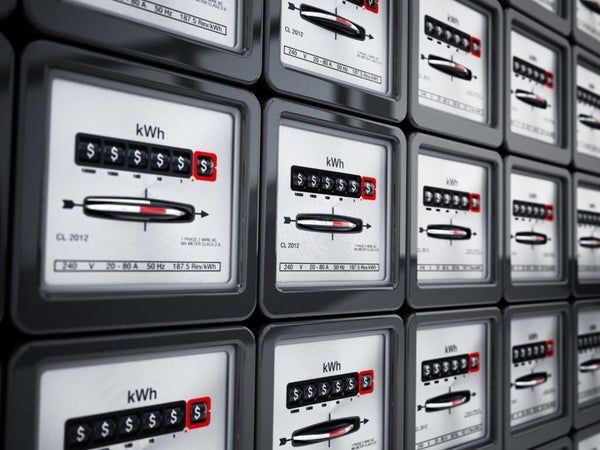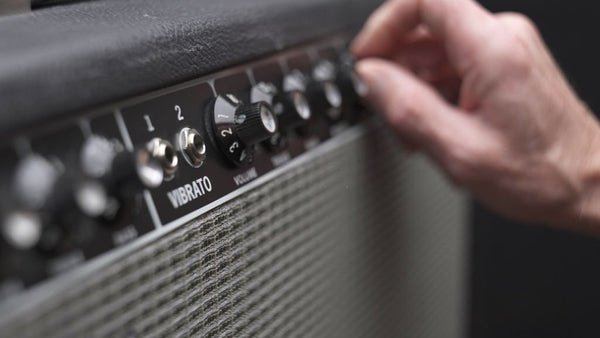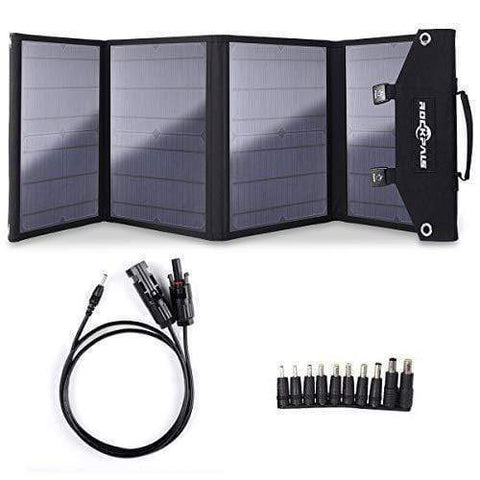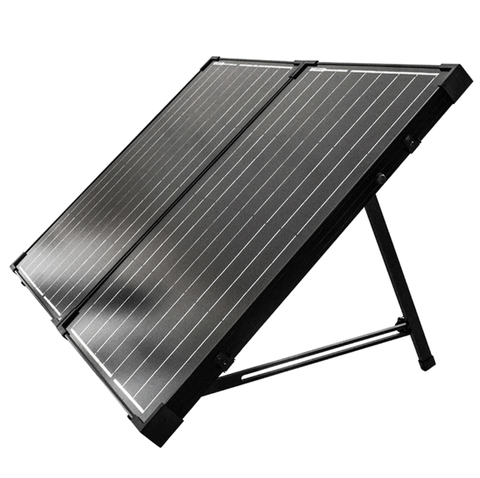kWh to Amp Calculator - Conversion Through Calculation - ShopSolar.com

# kWh to Amp Calculator

You’re going to encounter many calculators when dealing with solar and electrical systems that may seem overwhelming at first. Trying to figure out how to convert amps to kWh can seem like an exercise in futility.

The best approach is to break down what you have and what you need. That’s exactly what we’re here to do today.

Let’s go over how to use a kWh to amp calculator by looking at the two variables individually and their relationships with other units of power.

## What Are kWhWhether you’re using a watt usage calculator or simply comparing the sizes of different solar systems, kilowatts and kilowatt-hours will always be a part of the equation.

Another common place you’ll find this unit of power is on your monthly electricity bill.

Watts are the common unit of measure when we talk about electrical power.

The power requirements of your various appliances are generally labeled somewhere on the back and tell you both how much power you need to run them properly and what the consumption of each is over time.

In metric terms, a ‘Kilo’ is equivalent to 1,000 of what that unit is measuring. So a kilowatt is 1000 W, which makes it easy to convert kW to watts on the fly.

So, when we deal with watts and kilowatts, we refer to power. When we bring kilowatt hours into the conversation, we’re now referring to energy.

A good place to look to understand this is your monthly electric bill. To see how much energy you’ve used, you need to know how long a specific appliance is consuming energy.

This means we can describe kWh as power over some period.

When energy is measured in a household, we use kilowatt hours to keep the numbers relatively small.

So, when we use a kW to kWh calculator to figure out how much energy all the appliances in our homes are using, we’re adding time as a variable to obtain energy from power.Before we can investigate the current and amperes of a circuit, we need to understand the charge.

Charge is the property of electrons and protons that affects how they behave when they interact with each other. Opposite charges attract each other, whereas like charges repel.

Current is the flow of charge in a system or circuit and is measured in amperes.

Amps can be calculated if we know the voltage and resistance in a circuit, or they can be measured using an amp meter.

The flow of negative electrons through a circuit is called actual electron flow. For electrical equations, we refer to the positive charge moving down the wire known as conventional current.

No one knew about the existence of electrons and protons in the 1800s, so theories were made to say that electrons flowed from the positive to negative ends of a battery.

However, as time went on and further experiments were done, we discovered current traveled from the negative to positive ends of the circuit.

From a mathematical perspective, it doesn’t matter as the equations are all constants and don’t rely on the direction of the electrons.

Before we can dive into how to use a kWh to amp calculator, we need to understand the kW to amp calculator and work backward from there.

Once we’ve understood that, we can add hours or time as a variable to our equation.

## kWh to Amps Calculator in DC Circuit

For this example, let’s say we have a portable solar panel setup for our RV adventures.

This calculator uses a formula that states the current equals the kilowatts of your portable solar panels for camping multiplied by 1,000, divided by the voltage of the system.kWh = (W x 1,000) / V

If we want to convert the kilowatt hours to amps using this formula, we can either multiply the numerator by the amount of time or remove the hours from the kWh value.

## kWh to Amps in Single-Phase AC Circuits

Single-phase circuits contain only one power wire (phase) and one neutral wire. Both wires have current flowing between them.

This calculator uses a similar formula to the dc circuit formula but incorporates power factor into the denominator. The power factor is the ratio of working power to apparent power.

The former is measured in kW and the latter in kVA. You’ll often see power factor being a result of using a calculator that can convert kWh to kVA and then multiply it by time.

Again, we can either keep the kWh as a variable or remove the hours from the variable to reach our final current.## kWh to Amps in Three-Phase AC Circuit

Our final approach to using a kWh to amps calculator is in a three-phase circuit. For this formula, we’re dealing with circuits that have 3 power wires carrying the load between them.

The formula is the same as the previous ones, but we’re incorporating a constant of 1.73 in the denominator (approximately the square root of 3).

For all these formulae, we include hours as the time variable. This is because the current is based on hours and not at a constant or general rate.

## Conclusion

There isn’t a single, straightforward way of converting kilowatt-hours to amperes. The best approach is to convert kW to amps and then factor in time as a variable.

Despite there not being a dedicated calculator for the conversion, as long as you understand how these variables are related, you’re sure to find a way.

Did You Find Our Blog Helpful? Then Consider Checking: